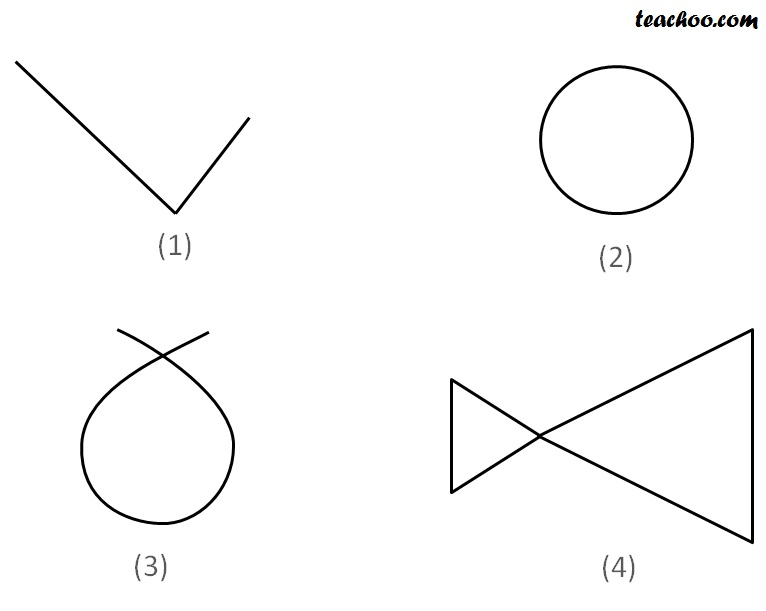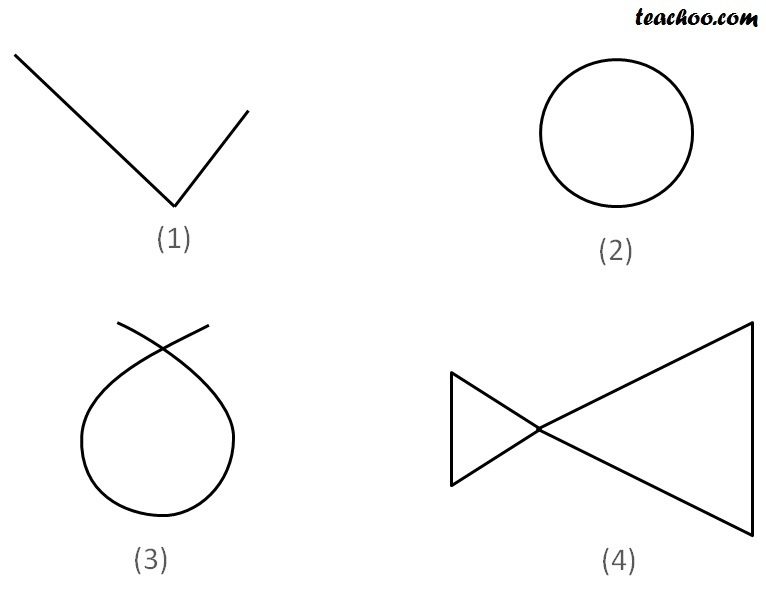Any line is a curve. Line can be straight or bended

Curves are formed by drawing without lifting the pen from the paper#### Simple Curve

A curve which does not cross itself is a simple curve.Here,

(1) & (2) are simple curves

(3) & (4) are not simple curves

#### Closed Curve

A curve which has no open ends is a closed curveHere,

(2) & (4) are closed curves

(1) & (3) are not closed curves

1. Chapter 4 Class 6 Basic Geometrical Ideas
2. Concept wise
3. Curve, Polygons